Polar representation

Chapter 5 Class 11 Complex Numbers
Concept wise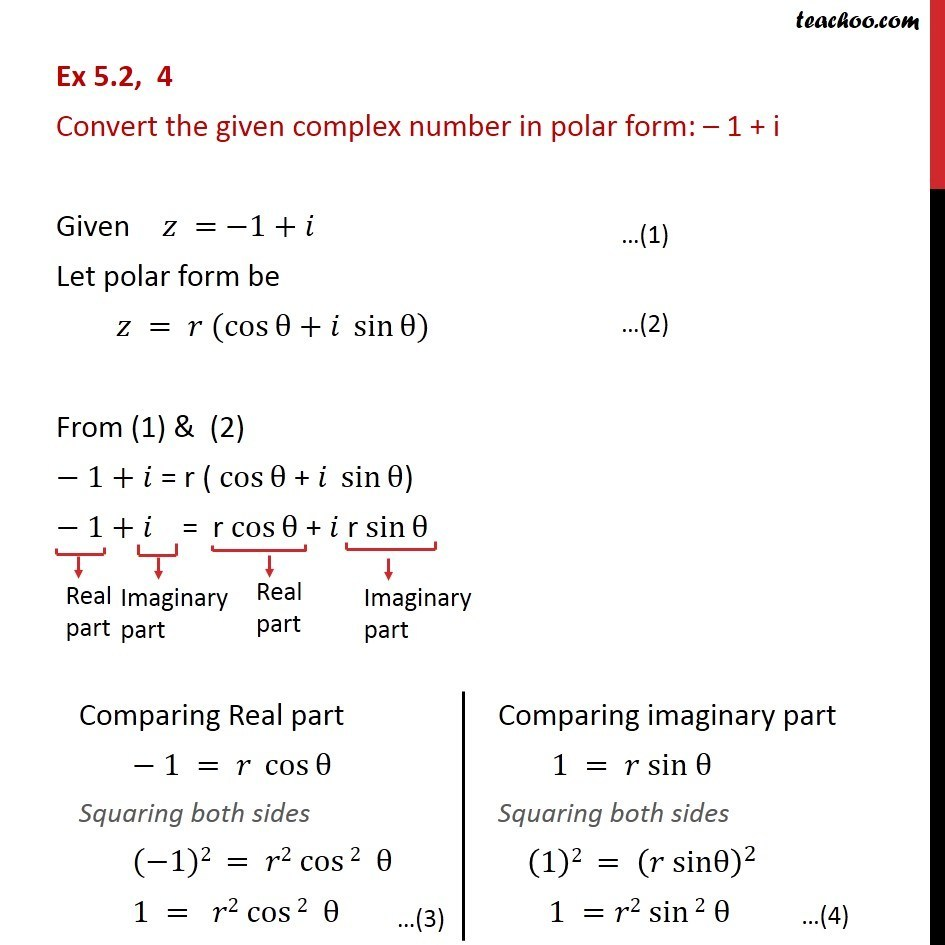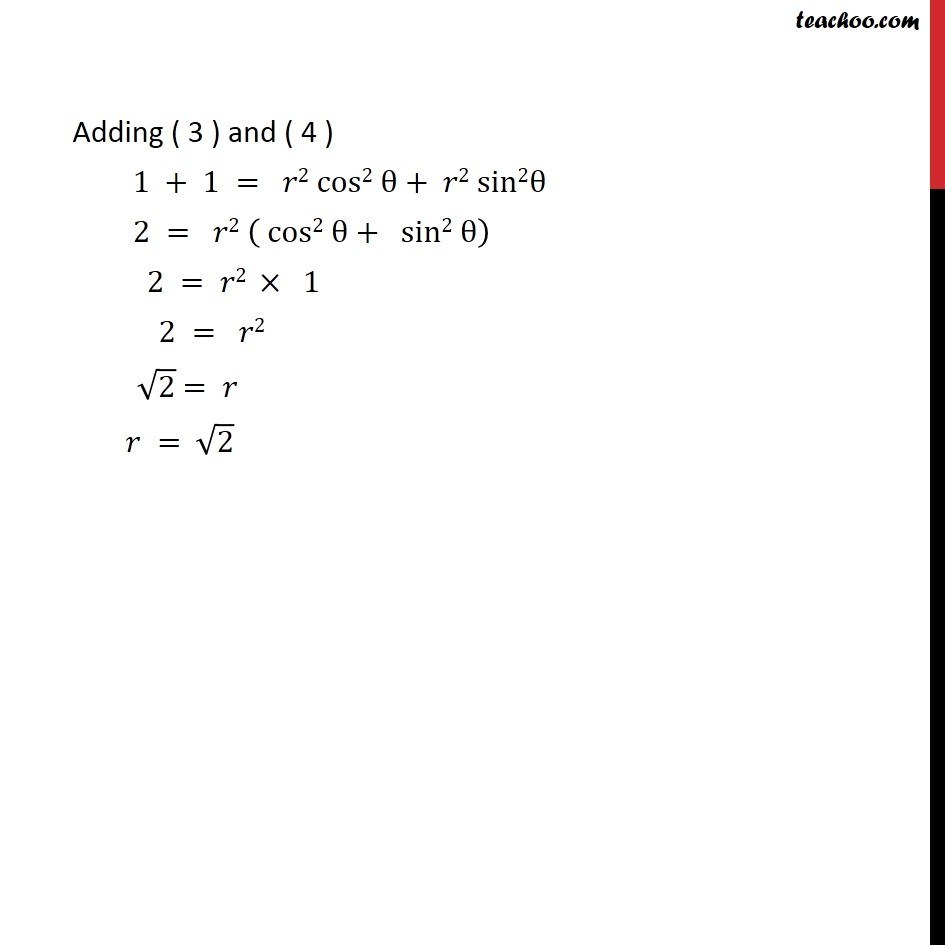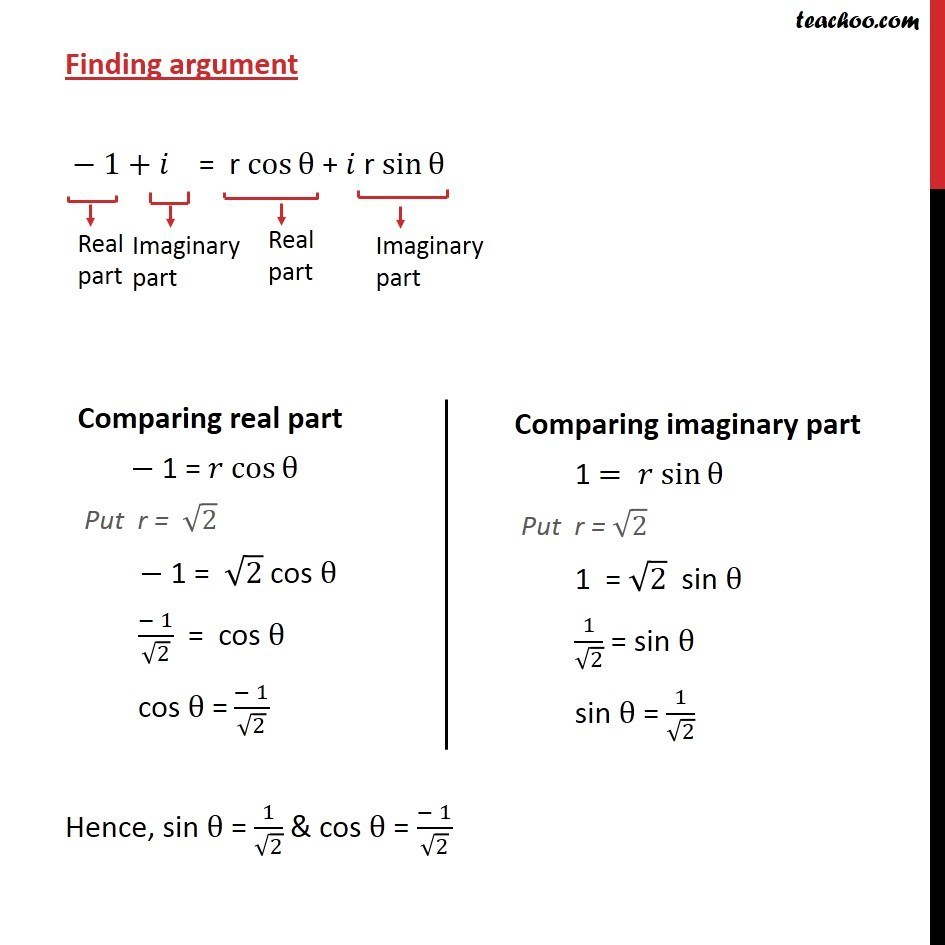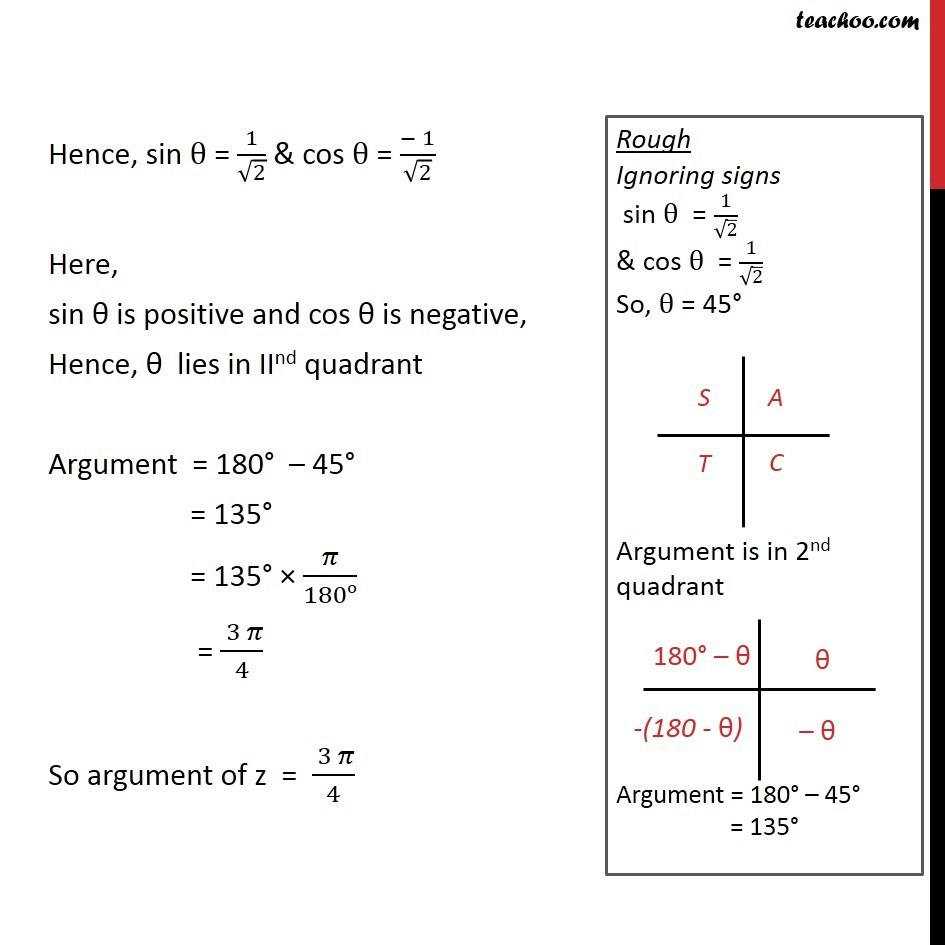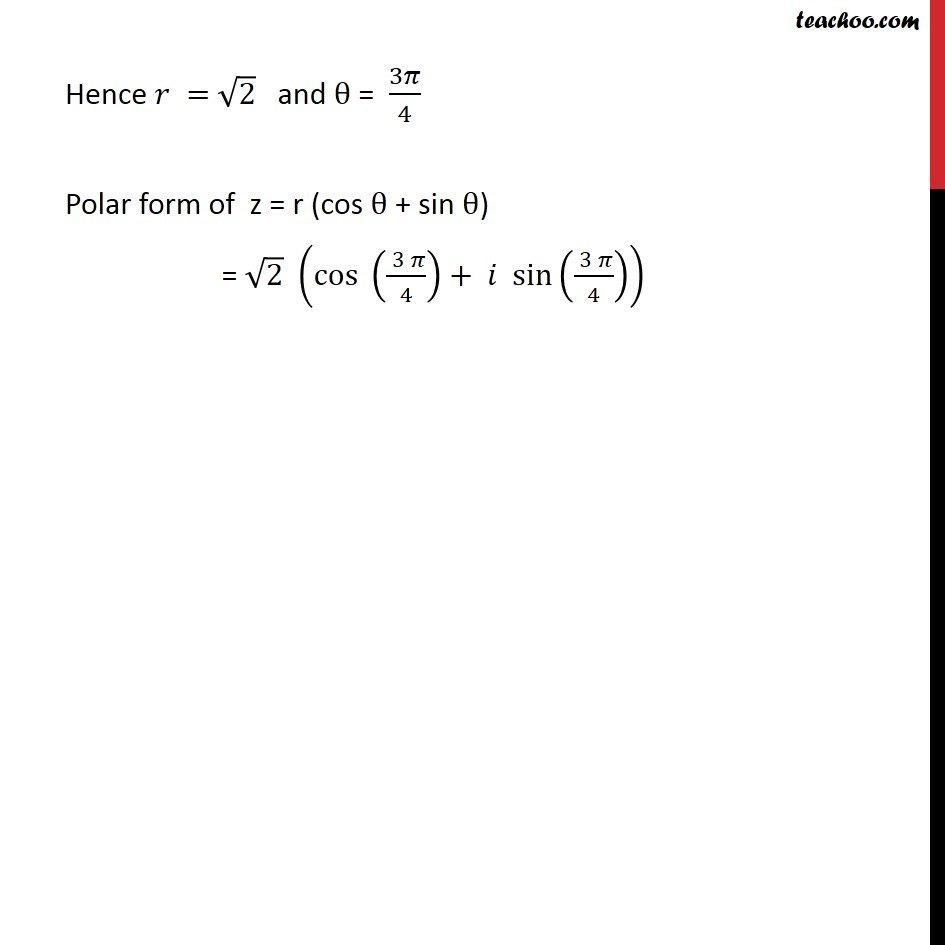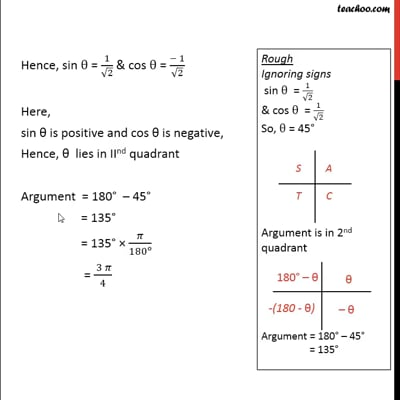This video is only available for Teachoo black users

Introducing your new favourite teacher - Teachoo Black, at only ₹83 per month

### Transcript

Ex 5.2, 4 Convert the given complex number in polar form: – 1 + i Given 𝑧 = −1+ 𝑖 Let polar form be 〖𝑧 = 𝑟 (cos〗⁡θ+𝑖 sin⁡θ) From (1) & (2) − 1+ 𝑖 = r ( cos⁡θ + 𝑖 sin⁡θ) − 1+ 𝑖 = r〖 cos〗⁡θ + 𝑖 r sin⁡θ Adding ( 3 ) and ( 4 ) 1 + 1 = 𝑟2 cos2 θ+ 𝑟2 sin2θ 2 = 𝑟2 ( cos2 θ+ sin2 θ) 2 = 𝑟2 × 1 2 = 𝑟2 √2 = 𝑟 𝑟 = √2 Finding argument − 1+ 𝑖 = r〖 cos〗⁡θ + 𝑖 r sin⁡θ Hence, sin θ = 1/√2 & cos θ = (− 1)/√2 Hence, sin θ = 1/√2 & cos θ = (− 1)/√2 Here, sin θ is positive and cos θ is negative, Hence, θ lies in IInd quadrant Argument = 180° – 45° = 135° = 135° × 𝜋/180o = ( 3 𝜋)/4 So argument of z = ( 3 𝜋)/4 Hence 𝑟 = √2 and θ = 3𝜋/4 Polar form of z = r (cos θ + sin θ) = √2 (cos (( 3 𝜋)/4)+ 𝑖 sin(( 3 𝜋)/4))